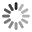The best jokes and joke writers!## Number Compliment

Q: What did the number zero say to the number eight?

A: "Nice belt."

## Dollars Equals Cents

Theorem: 1\$ = 1c. Proof: And another that gives you a sense of money disappearing. 1\$ = 100c= (10c)^2= (0.1\$)^2= 0.01\$= 1c.

Here \$ means dollars and c means cents. This one is scary in that I have seen PhD's in math who were unable to see what was wrong with this one. Actually I am crossposting this to sci.physics because I think that the latter makes a very nice introduction to the importance of keeping track of your dimensions.

## Kid Math

Mom + Dad - Rubber = U

## Circle Facts

Q: What's the difference between a radius and a diameter?